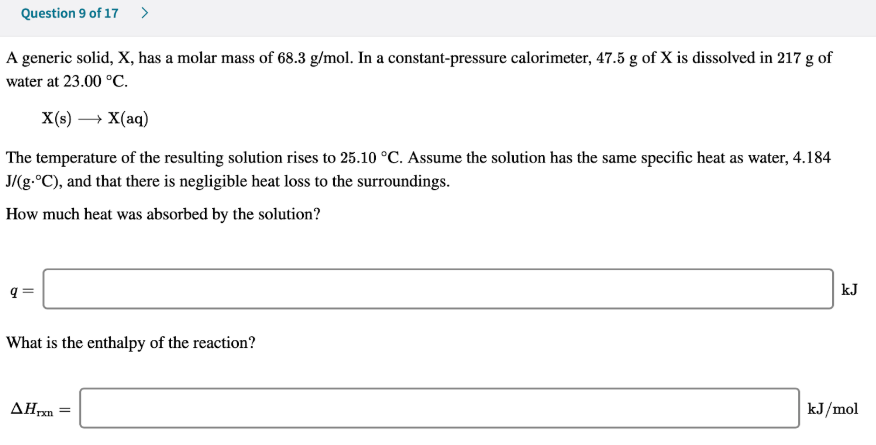# A generic solid, X, has a molar mass of 68.3 g/mol. In a constant-pressure calorimeter, 47.5 g of X is dissolved in 217 g of water at 23.00 °C. X(s) → X(aq) The temperature of the resulting solution rises to 25 °C. Assume the solution has the same specific heat as water, 4.184 J/(g•°C), and that there is negligible heat loss to the surroundings. How much heat was absorbed by the solution? What is the enthalpy of the reaction?